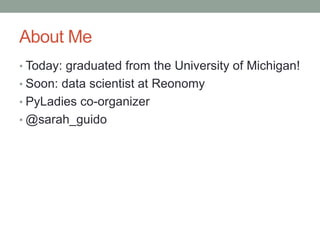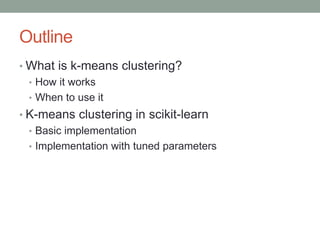Successfully reported this slideshow.

# K-means Clustering with Scikit-Learn×

# K-means Clustering with Scikit-Learn

Given at PyDataSV 2014

In machine learning, clustering is a good way to explore your data and pull out patterns and relationships. Scikit-learn has some great clustering functionality, including the k-means clustering algorithm, which is among the easiest to understand. Let's take an in-depth look at k-means clustering and how to use it. This mini-tutorial/talk will cover what sort of problems k-means clustering is good at solving, how the algorithm works, how to choose k, how to tune the algorithm's parameters, and how to implement it on a set of data.

Given at PyDataSV 2014

In machine learning, clustering is a good way to explore your data and pull out patterns and relationships. Scikit-learn has some great clustering functionality, including the k-means clustering algorithm, which is among the easiest to understand. Let's take an in-depth look at k-means clustering and how to use it. This mini-tutorial/talk will cover what sort of problems k-means clustering is good at solving, how the algorithm works, how to choose k, how to tune the algorithm's parameters, and how to implement it on a set of data.

### K-means Clustering with Scikit-Learn

1. 1. K-Means Clustering with Scikit-Learn Sarah Guido PyData SV 2014
2. 2. About Me • Today: graduated from the University of Michigan! • Soon: data scientist at Reonomy • PyLadies co-organizer • @sarah_guido
3. 3. Outline • What is k-means clustering? • How it works • When to use it • K-means clustering in scikit-learn • Basic implementation • Implementation with tuned parameters
4. 4. Clustering • Unsupervised learning • Unlabeled data • Split observations into groups • Distance between data points • Exploring the data
5. 5. K-means clustering • Formally: a method of vector quantization • Partition space into Voronoi cells • Separate samples into n groups of equal variance • Uses the Euclidean distance metric
6. 6. K-means clustering • Iterative refinement • Three basic steps • Step 1: Choose k • Iterate over: • Step 2: Assignment • Step 3: Update • Repeats until convergence has been reached
7. 7. K-means clustering • Assignment • Update
8. 8. K-means clustering • Advantages • Scales well • Efficient • Will always converge • Disadvantages • Choosing the wrong k • Convergence to local minimum
9. 9. K-means clustering • When to use • Normally distributed data • Large number of samples • Not too many clusters • Distance can be measured in a linear fashion
10. 10. Scikit-Learn • Machine learning module • Open-source • Built-in datasets • Good resources for learning
11. 11. Scikit-Learn • Model = EstimatorObject() • Unsupervised: • Model.fit(dataset.data) • dataset.data = dataset • Supervised would use the labels as a second parameter
12. 12. K-means in scikit-learn • Efficient and fast • You: pick n clusters, kmeans: finds n initial centroids • Run clustering jobs in parallel
13. 13. Dataset • University of California Machine Learning Repository • Individual household power consumption
14. 14. K-means in scikit-learn
15. 15. K-means in scikit-learn • Results
16. 16. K-means parameters • n_clusters • max_iter • n_init • init • precompute_distances • tol • n_jobs • random_state
17. 17. n_clusters: choosing k • Graphing the variance • Information criterion • Cross-validation
18. 18. n_clusters: choosing k • Graphing the variance • from scipy.spatial.distance import cdist, pdist • cdist: distance computation between sets of observations • pdist: pairwise distances between observations in the same set
19. 19. n_clusters: choosing k • Graphing the variance
20. 20. n_clusters: choosing k • Graphing the variance
21. 21. n_clusters: choosing k • Graphing the variance
22. 22. n_clusters: choosing k n_clusters = 4 n_clusters = 7
23. 23. n_clusters: choosing k • n_clusters = 8 (default)
24. 24. init • k-means++ • Default • Selects initial clusters in a way that speeds up convergence • random • Choose k rows at random for initial centroids • Ndarray that gives initial centers • (n_clusters, n_features)
25. 25. K-means revised • Set n_clusters • 7, 8 • Set init • kmeans++, random
26. 26. K-means revised n_clusters = 8, init = kmeans++ n_clusters = 8, init = random
27. 27. K-means revised n_clusters = 7, init = kmeans++ n_clusters = 7, init = random
28. 28. Comparing results: silhouette score • Silhouette coefficient • No ground truth • Mean distance between an observation and all other points in its cluster • Mean distance between an observation and all other points in the next nearest cluster • Silhouette score in scikit-learn • Mean of silhouette coefficient for all of the observations • Closer to 1, the better the fit • Large dataset == long time
29. 29. Comparing results: silhouette score • n_clusters=8, init=kmeans++ • 0.8117 • n_clusters=8, init=random • 0.6511 • n_clusters=7, init=kmeans++ • 0.7719 • n_clusters=7, init=random • 0.7037
30. 30. What does this tell us? • Patterns exist • Groups of similar observations exist • Sometimes, the defaults work • We need more exploration!
31. 31. A few tips • Clustering is a good way to explore your data • Intuition fails in high dimensions • Use dimensionality reduction • Combine with other models • Know your data
32. 32. Materials and resources • Scikit-learn documentation • scikit-learn.org/stable/documentation.html • Datasets • http://archive.ics.uci.edu/ml/datasets.html • Mldata.org • Blogs • http://datasciencelab.wordpress.com/